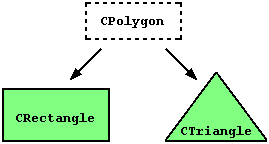# C++ 类之间的关系

## 友元函数(Friend functions)

```// friend functions
#include <iostream.h>

class CRectangle {
int width, height;
public:
void set_values (int, int);
int area (void) {return (width * height);}
friend CRectangle duplicate (CRectangle);
};

void CRectangle::set_values (int a, int b) {
width = a;
height = b;
}

CRectangle duplicate (CRectangle rectparam) {
CRectangle rectres;
rectres.width = rectparam.width*2;
rectres.height = rectparam.height*2;
return (rectres);
}

int main () {
CRectangle rect, rectb;
rect.set_values (2,3);
rectb = duplicate (rect);
cout << rectb.area();
}```

24

friend 函数可以被用来实现两个不同class之间的操作。广义来说，使用friend 函数是面向对象编程之外的方法，因此，如果可能，应尽量使用class的成员函数来完成这些操作。比如在以上的例子中，将函数duplicate() 集成在class CRectangle 可以使程序更短。

## 友元类 (Friend classes)

```// friend class
#include <iostream.h>

class CSquare;

class CRectangle {
int width, height;
public:
int area (void) {return (width * height);}
void convert (CSquare a);
};

Class CSquare {
private:
int side;
public:
void set_side (int a){side=a;}
friend class CRectangle;
};

void CRectangle::convert (CSquare a) {
width = a.side;
height = a.side;
}

int main () {
CSquare sqr;
CRectangle rect;
sqr.set_side(4);
rect.convert(sqr);
cout << rect.area();
return 0;
}```

16

## 类之间的继承(Inheritance between classes)class derived_class_name: public base_class_name;

```// derived classes
#include <iostream.h>

Class CPolygon {
protected:
int width, height;
public:
void set_values (int a, int b) { width=a; height=b;}
};

class CRectangle: public CPolygon {
public:
int area (void){ return (width * height); }
};

class CTriangle: public CPolygon {
public:
int area (void){ return (width * height / 2); }
};

int main () {
CRectangle rect;
CTriangle trgl;
rect.set_values (4,5);
trgl.set_values (4,5);
cout << rect.area() << endl;
cout << trgl.area() << endl;
return 0;
}```

20
10

```CPolygon::width           // protected access
CRectangle::width         // protected access
CPolygon::set_values()    // public access
CRectangle::set_values()  // public access```

class CRectangle: public CPolygon;

class daughter: protected mother;

## 什么是从基类中继承的? (What is inherited from the base class?)

• 构造函数Constructor 和析构函数destructor
• operator=() 成员
• friends

derived_class_name (parameters) : base_class_name (parameters) {}

```// constructors and derivated classes
#include <iostream.h>

class mother {
public:
mother ()
{ cout << "mother: no parameters\n"; }
mother (int a)
{ cout << "mother: int parameter\n"; }
};

class daughter : public mother {
public:
daughter (int a)
{ cout << "daughter: int parameter\n\n"; }
};

class son : public mother {
public:
son (int a) : mother (a)
{ cout << "son: int parameter\n\n"; }
};

int main () {
daughter cynthia (1);
son daniel(1);
return 0;
}```

mother: no parameters
daughter: int parameter

mother: int parameter
son: int parameter

```daughter (int a)          // 没有特别制定：调用默认constructor
son (int a) : mother (a)  // 指定了constructor: 调用被指定的构造函数```

## 多重继承(Multiple inheritance)

class CRectangle: public CPolygon, public COutput {
class CTriangle: public CPolygon, public COutput {

```// multiple inheritance
#include <iostream.h>

class CPolygon {
protected:
int width, height;
public:
void set_values (int a, int b)
{ width=a; height=b;}
};

class COutput {
public:
void output (int i);
};

void COutput::output (int i) {
cout << i << endl;
}

class CRectangle: public CPolygon, public COutput {
public:
int area (void)
{ return (width * height); }
};

class CTriangle: public CPolygon, public COutput {
public:
int area (void)
{ return (width * height / 2); }
};

int main () {
CRectangle rect;
CTriangle trgl;
rect.set_values (4,5);
trgl.set_values (4,5);
rect.output (rect.area());
trgl.output (trgl.area());
return 0;
}```

20
10